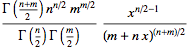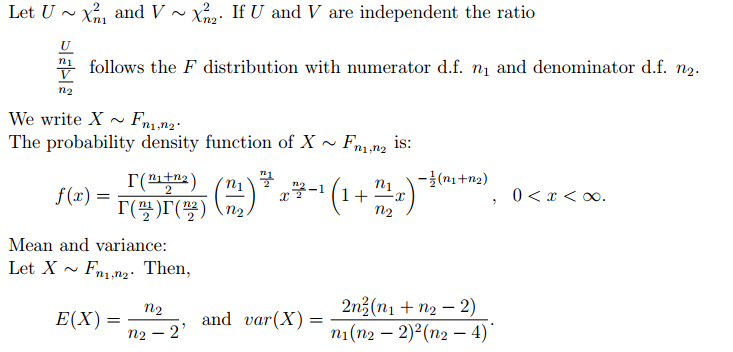$X = \frac{U_1/d_1}{U_2/d_2}$
will have F-distribution (with parameters d1 and d2)

where

With a density of:whereis the gamma function.Proof:

Larsen, R. J., & Marx, M. L. (2010). Introduction to Mathematical Statistics and Its Applications (5 edition.). Boston: Pearson.
and

http://webpages.math.luc.edu/~jdg/w3teaching/stat_305/sp06/pdf/densityF.pdf